• 2021-04-19 11:41:36

点击查看jqdata sdk 详细内容

注册登录

策略

新建股票策略

填入下面代码：

利用凯利公式进行投资测试，基准为沪深300

def initialize(context):
g.stock='000300.XSHG'
g.N=60
g.high=0
g.drawdown=0.4

set_benchmark('000300.XSHG')
set_option('use_real_price',True)
log.set_level('order','error')

def handle_data(context,data):
g.high=max(g.high,context.portfolio.total_value)
close=list(attribute_history(g.stock, g.N, '1d', 'close').close)
v=[(s2/s1) for (s1,s2) in zip(close[:-1],close[1:])]
print(v)
m=mean(v)
s=np.std(v)
mu=np.log(m)
sigma2=np.log(s**2/m**2 + 1)
#f_star=np.abs(mu/sigma2)  ##取绝对值
f_star=mu/sigma2
#print("m is %s " % m)
#print("f_star is %s " % f_star)
init_value = context.portfolio.total_value*g.drawdown*f_star*0.5
# print("*****************"*3)
# print(init_value)
print("---"*20)
print(f_star)

if m>0:
order_target_value(g.stock,init_value)
else:
order_target_value(g.stock,0)



然后选择日期，金额 100000

然后就可以回测了

不提供任何投资建议，学习研究用

希望对大家有所帮助

你会发现线上数据有局限性，不如本地运行快等，建议选择本地数据

更多相关内容
• 37-量化策略回测：创建属于自己的回测框架 38-量化策略回测：如何利用聚宽平台回测交易策略【本次加推篇】 39-量化策略回测：基于BackTrader建立双均线策略 40-远程下单方案：微信机器人实时提醒交易 41-远程下单...

导览

本文为掘金小册课程加推篇！小册还在持续加推中，敬请期待！！！目前已经加推20节！！！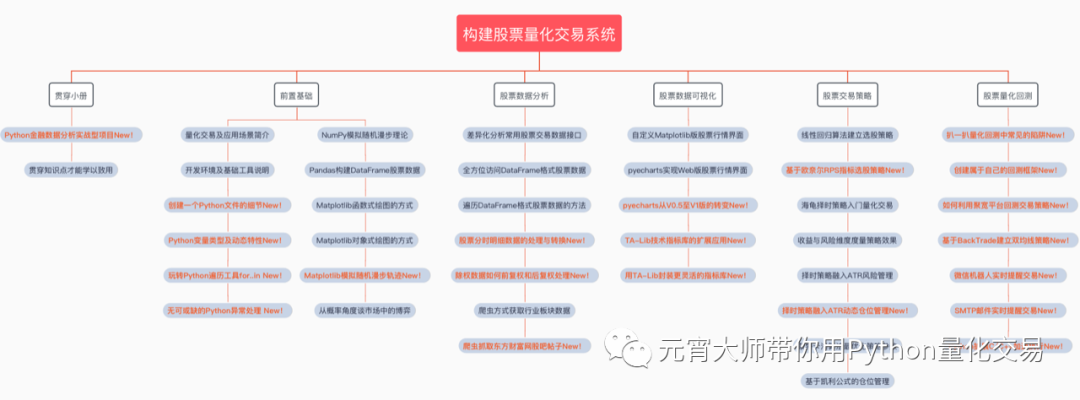超值的43节课程！从目录就能看出来！

# 43-结尾篇：贯穿知识点才能学以致用

前言

我们经过了自己动手搭建量化回测框架这个过程之后，能够对回测有一定的认识。

接下来可以探索下那些现有的回测框架或者平台，试着找到适合自己风格的方式。

这里我们选一个比较普及的线上回测平台——聚宽，通过几个简单的例程来熟悉下这个平台的使用特点。

编程框架

首先文件中要导入jqdata和其他使用到的库。如下所示：

# 导入函数库
import jqdata

import pandas as pd
import numpy as np
import talib as tl

基本的框架可以概括为“初始化+周期循环”框架。

首先建立一个初始化函数initialize，在函数里面可以设置基准、滑点、手续费等初始化操作。

然后选择run_daily/run_weekly/run_monthly中的一种周期去循环执行策略。

比如官网上最简单的一个例程，如下所示：

# 导入聚宽函数库
import jqdata
# 初始化函数，设定要操作的股票、基准等等def initialize(context):
# 定义一个全局变量, 保存要操作的股票
# 000001(股票:平安银行)
g.security = '000001.XSHE'
# 运行函数
run_daily(market_open, time='every_bar')
if g.security not in context.portfolio.positions:
order(g.security, 1000)
else:
order(g.security, -800)


这个例程里先在初始化函数 initialize里指定操作'000001.XSHE'平安银行，然后就是每天调用market_open函数，每次调用时候判断没有股票时就买入1000股,有股票时卖出800股……虽然是一个很没意义的策略，但是对理解如何在聚宽上写策略还是比较直观的。

大家在编写策略的时候是要使用聚宽上封装了的API函数的，可以查看官网的介绍。比如set_benchmark设置基准、set_order_cost设置佣金/印花税、set_slippage设置滑点、use_real_price设置动态复权(真实价格)模式、order按股数下单、get_ticks获取tick数据等等，不得不说从这方面来看使用上还是蛮方便的。

单股票双均线择时策略

我们拿双均线策略这个入门的策略来回测一下，M5上穿M10时达到一定幅度时买入，M5下穿M10时卖出。

在初始化函数中设定操作的股票、基准、开启动态复权模式、设定每天调用的market_open函数。

接下来策略的逻辑实现都在market_open函数中。

先得到M5和M10的均线数值，attribute_history函数得到的是最近N日的收盘价数据，以close_data_5为例，每天会返回如下数值（每天都会执行一次函数，返回N日的收盘价），然后求平均即为MA5。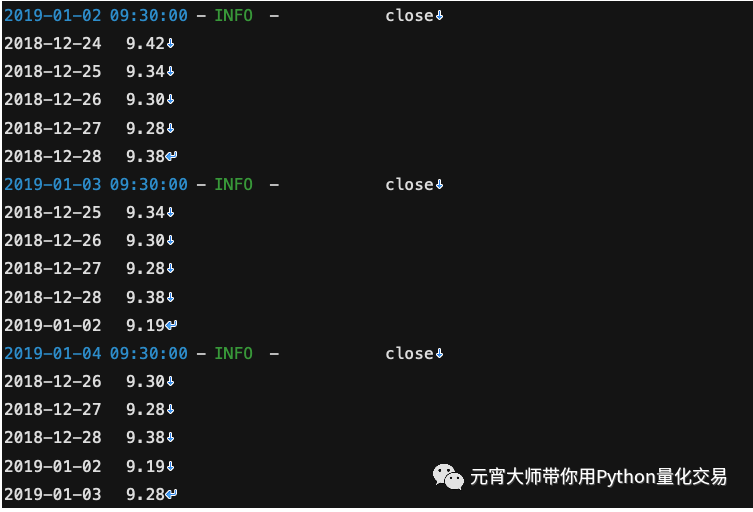剩下的就是买卖点的逻辑判断，比如出现金叉买入、出现死叉卖出之类的。最后record函数还可以选择绘制收盘价、均线的走势。

整体代码我们会更新到掘金小册课程。

回测界面如下所示：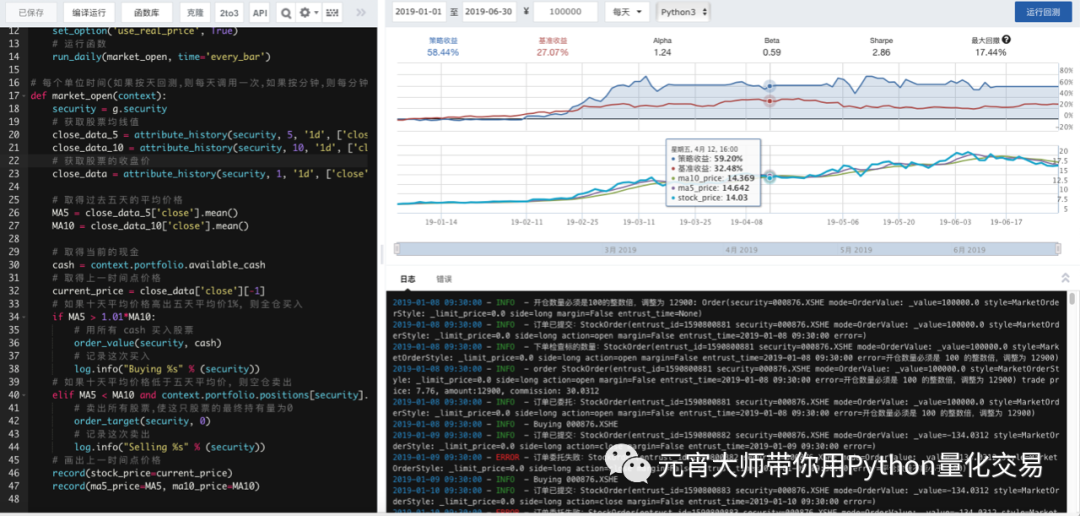多股票双均线择时策略

很多时候我们买股票都是买多只股票的，那么如何回测多只股票情况下的双均线择时策略呢？

比如当某只股票M5上穿M10时达到一定幅度时买入100股，M5下穿M10时则卖出。

其实主要更改在于建立一个股票池，如下所示：

# 设置我们要操作的股票池
g.stocks = ['000001.XSHE','000002.XSHE','000004.XSHE','000005.XSHE']


然后在每次调用交易执行中不断去循环判断这几个股票是否达到交易条件。

回测界面如下所示：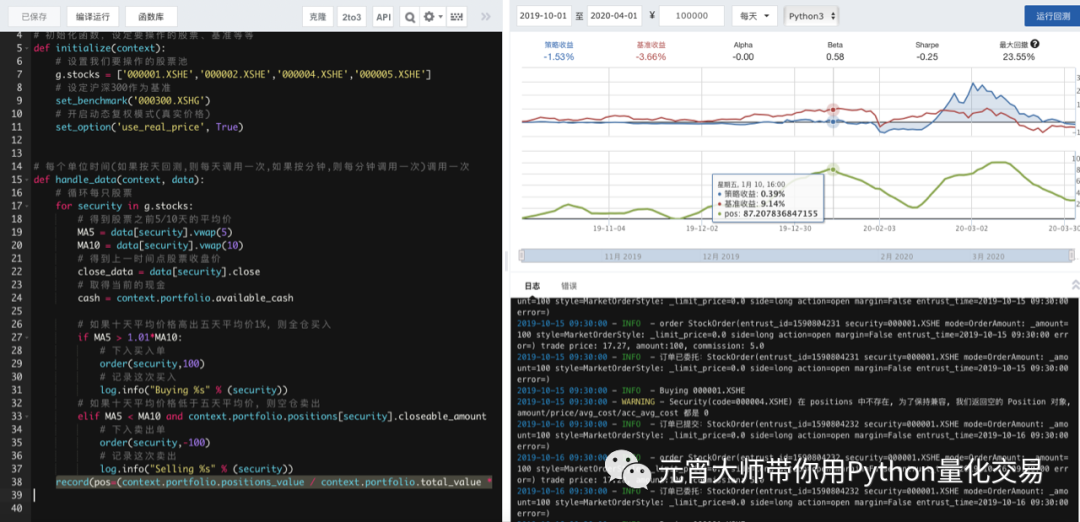总结

聚宽平台给量化交易者提供了一个很不错的平台，使用过程中，我的感觉是关于内置的API函数还是比较灵活的，特别是与数据相关的接口。前提是要非常熟悉这些接口的特点和使用方法。

不过回测过程中速度确实有点慢，编写和调试代码的环境和我本地相比是有点不太灵活。

总体来说瑕不掩瑜吧，大家使用后有什么想法吗？可以在留言区谈谈。

代码已更新至掘金小册，请查收！

更多量化交易内容可扫码以下课程！—————————————————海报未更新，直接扫码！

扫码加入星球！
一起搭建属于自己的交易系统
—————————————————

微信关注：‘元宵大师带你用Python量化交易’


展开全文• 数据接口使用的是聚宽量化平台的API。 import numpy as np import datetime import jqdata import pandas as pd import matplotlib.pyplot as plt import json #字符转换 import matplotlib.ticker as mtk import ...

本科时代自己撸的一套简易股票量化回测框架，供新手入门学习研究。支持不同标的、多参数的批量回测，用于快速开发迭代交易策略。
数据接口使用的是聚宽量化平台的API。

import numpy as np
import datetime
import jqdata
import pandas as pd
import matplotlib.pyplot as plt
import json           #字符转换
import matplotlib.ticker as mtk
import math
import statsmodels.tsa.ar_model as sm
import scipy.signal as signal
from scipy.interpolate import interp1d
import talib

matplotlib.rcParams['axes.unicode_minus']=False  #图像中负号显示不正常


策略部分：

def Trading_strategy(i,code,date,current_date,current_price,Highprice,position,open_price,ATRstop,FilterTimes,Window,fee,ROR,ROR_open):
####策略参数####

price = get_price(code, end_date = date, frequency='1d', fields=('close','high','low','open'),count= 40)
KAMA = talib.KAMA(price['close'],Window)
ATR = talib.ATR(price['high'],price['low'],price['close'], Window)[-1]
Filter = talib.STDDEV(KAMA[-Window:],Window)[-1]

#平仓
if position == 1:
if KAMA[-1]-KAMA[-2] < -Filter*FilterTimes:
position,open_price,Highprice,ROR_open = Market_out(open_price,position,current_date,current_price,fee,ROR,ROR_open)
if position == -1:
if KAMA[-1]-KAMA[-2] > Filter*FilterTimes:
position,open_price,Highprice,ROR_open = Market_out(open_price,position,current_date,current_price,fee,ROR,ROR_open)

#开仓
if position == 0:
if KAMA[-1]-KAMA[-2] > Filter*FilterTimes:
position,open_price,Highprice = Market_in(current_date,code,1,current_price)
if KAMA[-1]-KAMA[-2] < -Filter*FilterTimes:
position,open_price,Highprice = Market_in(current_date,code,-1,current_price)

#追踪止损
if position > 0:
Highprice = max(Highprice,price['close'][-1])
if price['close'][-1] <= (Highprice - ATRstop*ATR):
#print ("追踪止损")
position,open_price,Highprice,ROR_open = Market_out(open_price,position,current_date,current_price,fee,ROR,ROR_open)

if position < 0:
Highprice = min(Highprice,price['close'][-1])
if price['close'][-1] >= (Highprice + ATRstop*ATR):
#print ("追踪止损")
position,open_price,Highprice,ROR_open = Market_out(open_price,position,current_date,current_price,fee,ROR,ROR_open)

return position,open_price,Highprice,ROR_open


回测功能函数：

#开仓函数
def Market_in(current_date,code,ratio,current_price):
open_price  = current_price
position = ratio
Highprice = current_price
print ("开仓",current_date,position,current_price)
return position,open_price,Highprice

#平仓函数
def Market_out(open_price,position,current_date,current_price,fee,ROR,ROR_open):
if open_price == 0:
ROR_single = 0
else:
if position > 0:
ROR_single = (current_price-open_price)/open_price
if position < 0:
ROR_single = -(current_price-open_price)/open_price

ROR = (1+ROR_open)*abs(position)*(ROR_single+1) + (1+ROR_open)*(1-abs(position)) -1

open_price = 0
position = 0
Highprice = 0

ROR_open = (ROR+1)*(1-fee) - 1
print ("平仓",current_date,position,current_price)
return position,open_price,Highprice,ROR_open

#计算年化收益
def annualized_return(return_list):

n = len(return_list)
ar = ((return_list[-1])**(250/n)-1)
return ar

#计算最大回撤
def MaxDrawdown(return_list):
Dlist = -(np.maximum.accumulate(return_list) - return_list) / np.maximum.accumulate(return_list)
i = np.argmax((np.maximum.accumulate(return_list) - return_list) / np.maximum.accumulate(return_list))
if i == 0:
return 0
j = np.argmax(return_list[:i])
return Dlist,(return_list[j] - return_list[i]) / (return_list[j])

#计算夏普比率
def sharpe_ratio(ar,day_ror):
return_stdev = np.std(day_ror)
sharpe_ratio = (ar - 0.04) / (np.sqrt(250)*return_stdev)
return sharpe_ratio

################################主回测过程#################################
def Retest(code,start,end,fee,ATRstop,FilterTimes,window):
####基本参数####
datelist = []                 #回测日期列表
Retest_data = get_price(code, start_date = start, end_date = end, frequency='1d', fields=('close'))['close']
datelist = Retest_data.index  #回测日期列表
open_flag = 0                 #开仓信号，1开多，-1开空，0平仓
ROR = 0                       #策略收益率
ROR_list = []                 #策略收益率序列
ROR_open = 0                  #每次开仓前的累计收益率
position = 0                  #持仓量
position_list = []            #持仓量序列
ROR_single = []               #单次持仓收益率
ROR_single_list = []          #单次持仓收益率序列
open_price = 0
ROR_open_record = 0
current_N = 0
Highprice = 0
HL = np.array(np.zeros(500),dtype=complex)
Dlist = []
Dmax = 0                      #计算最大回撤
profit_times = 0              #计算胜率和盈亏比
profit_price = 0
loss_times = 0
loss_price = 0

#开始回测
for i in range(len(datelist)):
#print (Retest_data.index[i])
if i > 0:
#每日数据
date = Retest_data.index[i]
current_date = Retest_data.index[i]
current_price = get_price(code, end_date = current_date, frequency='1d', fields=('close'),count= 2)['close'].values[-1]

if ROR_open_record != ROR_open:
if ROR_open_record > ROR_open:
loss_times += 1
loss_price += ROR_open - ROR_open_record
if ROR_open_record < ROR_open:
profit_times += 1
profit_price += ROR_open - ROR_open_record

ROR_open_record = ROR_open

#单次持仓收益率计算
if open_price == 0:
ROR_single = 0
else:
if position > 0:
ROR_single = (current_price-open_price)/open_price
if position < 0:
ROR_single = -(current_price-open_price)/open_price

ROR = (1+ROR_open)*abs(position)*(ROR_single+1) + (1+ROR_open)*(1-abs(position)) -1

position_list.append(position)
ROR_single_list.append(ROR_single)
ROR_list.append(ROR)

return_list = [i+1 for i in ROR_list]
ar = annualized_return(return_list)
Dlist,Dmax = MaxDrawdown(return_list)

Benchmark = (Retest_data-Retest_data)/Retest_data

day_ror = []                                 #策略每日收益率
for i in range(len(return_list)):
if i == 0 :
day_ror.append(0)
else:
day_ror.append(((return_list[i] - return_list[i-1])/return_list[i-1]))
sharpe_r = sharpe_ratio(ar,day_ror)

#收益率回撤图
fig = plt.figure(figsize=(15,18))

Dlist_draw = [x*100 for x in Dlist]
ax4.bar(datelist,Dlist_draw,label='drawdown',width = 5,color='c')
ax4.grid(True)
legend(loc='upper right')
#ax4.set_xlabel('Date',fontsize=15)
ax4.set_ylabel('Drawdown Ratio',fontsize=15)
fmt='%.2f%%'
yticks = mtk.FormatStrFormatter(fmt)
ax4.yaxis.set_major_formatter(yticks)

#回测收益率图

#fig, ax1 = plt.subplots(figsize=(12,6))
Benchmark_draw = [x*100 for x in Benchmark]
ROR_list_draw = [x*100 for x in ROR_list]
ax1.plot(datelist,Benchmark_draw,label='benchmark')
ax1.plot(datelist,ROR_list_draw,label='strategy')
ax1.grid(True)
legend(loc='upper right')
#ax1.set_xlabel('Date',fontsize=15)
ax1.set_ylabel('Rate of Return',fontsize=15)
fmt='%.2f%%'
yticks = mtk.FormatStrFormatter(fmt)
ax1.yaxis.set_major_formatter(yticks)

ROR1 = pd.DataFrame(ROR_list_draw)

#回测持仓图
#fig, ax2 = plt.subplots(figsize=(12,3))
ax2.plot(datelist,position_list,label='position', color='pink')
ax2.grid(True)
legend(loc='upper right')
ax2.set_xlabel('Date',fontsize=15)
ax2.set_ylabel('Position',fontsize=15)
plt.show()

#回测单次持仓盈亏图
fig, ax3 = plt.subplots(figsize=(12,3))
ax3.plot(datelist,ROR_single_list,label='rate of return')
ax3.grid(True)
legend(loc='upper left')
ax3.set_xlabel('Date',fontsize=15)
ax3.set_ylabel('ROR single time',fontsize=15)
plt.show()

return Benchmark[-1],ROR_list[-1],ar,Dmax,sharpe_r,profit_times,loss_times,profit_price,loss_price


执行函数：

import datetime
from dateutil.relativedelta import relativedelta

start = '2018-01-01 00:00:00'
end = '2019-01-01 00:00:00'

code = ['000016.XSHG','000300.XSHG','000905.XSHG']
# code = ['IF9999.CCFX']

# 批量回测参数组
x = 
y = [0.1]
z = 
####################
fee_list = [0.001]  #手续费+滑点，千一

for element in code:
for i in x:
for j in y:
for k in z:
for fee in fee_list:
print (i,j,k,f)
bm,tv,ar,Dmax,sharpe_r,profit_times,loss_times,profit_price,loss_price = Retest(element,start,end,fee,i,j,k)
print ("Strategy yield: %.2f%%"%(tv*100))
print ("Benchmark yield: %.2f%%"%(bm*100))
print ("Maximum drawdown ratio: %.2f%%"%(Dmax*100))
print ('盈利次数：%d, 亏损次数：%d'%(profit_times, loss_times))
print ('胜率: %f'%(profit_times/(profit_times+loss_times)))
print ('盈利金额（占初始金额比例）：%f, 亏损金额（占初始金额比例）：%f'%(profit_price, -loss_price))
print ('盈亏比: %f'%(-profit_price/loss_price))



输出：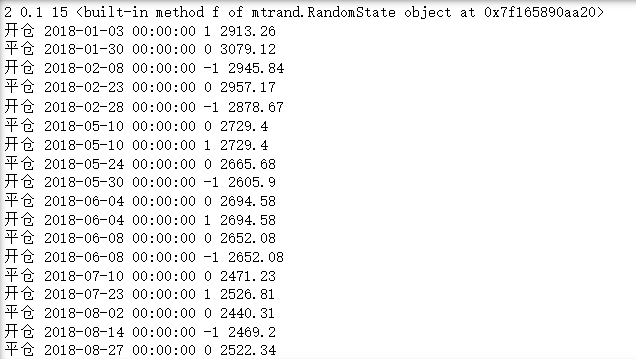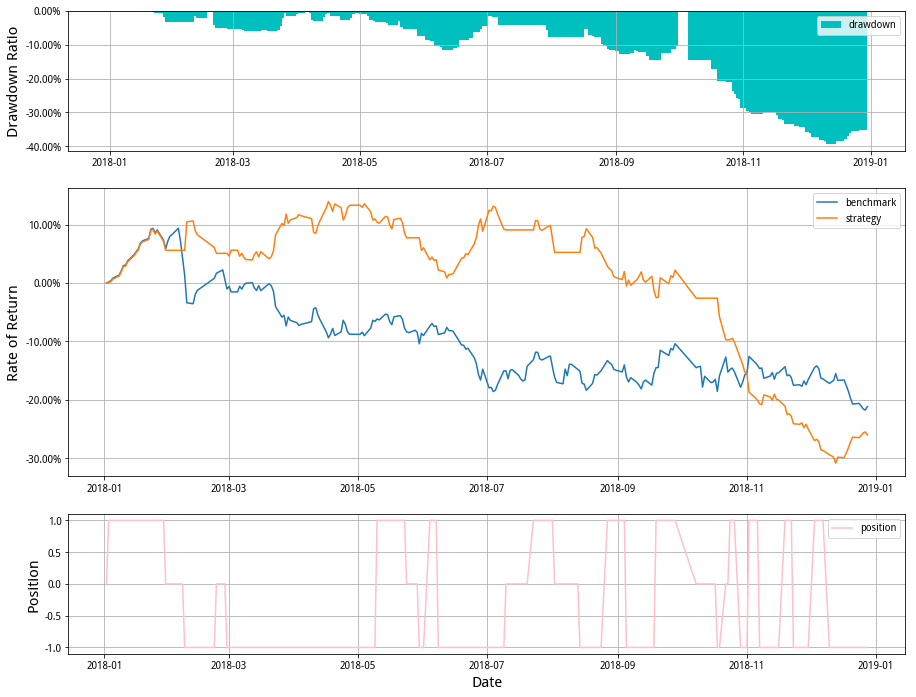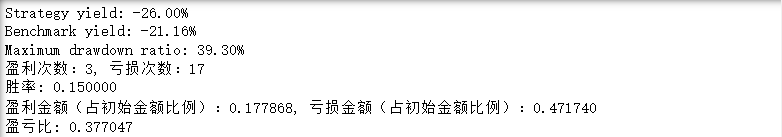展开全文python
• QUANT 聚宽joinQuant 官方文档API doc - プロノCodeSteel - CSDN博客 QUANT 量化交易常见概念解析 - プロノCodeSteel - CSDN博客 QUANT 基础知识之 未来公式的定义与使用 - プロノCodeSteel - CSDN...

### 在聚宽的API 描述的框架中  变量g用于保存全局变量

全局对象 g，用来存储用户的各类可被pickle.dumps函数序列化的全局数据

在模拟盘中，如果中途进程中断，我们会使用[pickle.dumps]序列化所有的g下面的变量内容, 保存到磁盘中，再启动的时候会反序列化，这样模拟盘就不会有任何数据影响。如果没有用g声明，会出现模拟盘重启后，变量数据丢失的问题。

如果不想 g 中的某个变量被序列化, 可以让变量以 '__' 开头, 这样, 这个变量在序列化时就会被忽略

def initialize(context):
g.security = "000001.XSHE"
g.count = 1
g.flag = 0

def process_initialize(context):
# 保存不能被序列化的对象, 进程每次重启都初始化, 更多信息, 请看 [process_initialize]
g.__q = query(valuation)

def handle_data(context, data):
log.info(g.security)
log.info(g.count)
log.info(g.flag)


展开全文• 1 概述Hello，大家好，我们准备收集并整理...回测或者模拟交易中的报错，可以在报错代码中找到“user_code.py”，然后再看下对应的报错在您代码中哪行，重点关注下最后一行报错提示，也可以直接将最后一行报错复制...
• 聚宽平台可以方便进行策略编写、投资研究、数据回测等各项功能，云端的python环境可以很好支持。不过使用下来也有些不便之处，比如代码调试困难，虚拟机内存1G限制等问题。有时候需要将数据倒到本地调试代码。下面...python
• 另外聚宽还提供了一个可以在本地Python开发环境中使用的jqfactor_analyzer，在本地直接用pip安装即可。二者大同小异。这里以JQFactor为例介绍其单因子分析的基本概念和分析结果的详细说明。 这是本文用到的示范代码...量化投资 Python
• 前言一个完整的量化交易系统离不开人机交互功能的应用，特别是在回测阶段，我们需要不断更改参数因子、更换交易策略以应对当前的市场。因此创建完整的、功能键全的GUI用户界面至关重要。前几期我们...
• 1、安装 ，在cmd下输入： pip install jqdatasdk，安装成功显示如下： 2、注册聚宽账号， ... 3、获取指定日期的新闻联播的文字新闻数据 ...df = finance.run_query(query(finance.CCTV_...聚宽，多因子分析有一点...python
• 【转】多因子策略探索（1...而聚宽平台的架构可以在定义的任意时点，操作市场上任意标的的仓位，非常适合研究/实盘一揽子标的的策略，笔者在这里就用聚宽框架展示几个横截面因子的回测。 期开始研究多因子策略，看了...python 开发语言 量化交易
• 最近需要搭建本地的量化回测平台来满足个性化的回测需求，填补聚宽、真格等在线回测平台的缺陷。之前没有做过相关的工作，所以打算先学习一下vn.py的回测模块的框架，读一下vn.py的源码。vnpy的源码可以从github上...
• 这里简要介绍一下如何利用聚宽免费数据进行量化分析： 1、使用聚宽的量化网站平台或金融终端： 聚宽的量化网站平台网址是：https://www.joinquant.com/ 聚宽的金融终端在这个主页上就可以看到下载链接，目前提供的...量化投资
• 量化策略入门系列文章是本人学习股票量化笔记，最终输出结果希望是一个可在本地运行的回测框架，包含数据获取，数据处理，策略回测等。 本文主要为了介绍如何利用聚宽(JoinQuant)的数据接口下载金融数据。主要针对量...python
• 进行股票量化回测，面临的第一个坑就是数据坑——首先你得有数据，才能进行下一步工作。 在股票数据坑中，有一个很大的坑叫做股票的复权价格。   如果一个股票公告说进行分红、配股或者送转，那么这只股票就被...python
• ## 聚宽JQData说明书

千次阅读 2019-02-19 19:50:26
JQData是聚宽数据团队专门为金融机构、学术团体和量化研究者们提供的本地量化金融数据服务。使用JQData，可快速查看和计算金融数据，无障碍解决本地、Web、金融终端调用数据的需求。历经3年沉淀，15万宽客及数百家...
• 前言一个完整的量化交易系统离不开人机交互功能的应用，特别是在回测阶段，我们需要不断更改参数因子、更换交易策略以应对当前的市场。因此创建完整的、功能键全的GUI用户界面至关重要。市面上也出...css html github javascript
• 目前聚宽已支持国内外50多家高校的教学使用，包括『本地数据支持』、『投研环境支持』、『线上线下量化课程』、『量化大赛』、『工作机会』等多元化的量化生态服务，助力学生们步步进阶。 01 | 前言 近年来，...
• 前言一个完整的量化交易系统离不开人机交互功能的应用，特别是在回测阶段，我们需要不断更改参数因子、更换交易策略以应对当前的市场。因此创建完整的、功能键全的GUI用户界面至关重要。我们在【搭...
• zipline是美国著名的量化策略平台quantopian开发和维护的量化交易库，并且quantopian量化平台的回测引擎也是基于zipline的，除此之外，像国内比较有名的三大矿聚宽(JointQuant)、米筐(RiceQuant)、优矿的回测引擎也...python
• 6款优秀量化交易回测框架！VNPY位居第一 一个策略从想法，到测试，在到实盘，然后改进，进入另一个循环，需要很多的时间和精力。这时候选择一款高效、灵活的测试系统就是当务之急了。即使最后你可能需要写自己的系统...python github java c语言 程序人生
• 数据库为：mongodb 数据获取包为：tushare(但是获取市净率需要... 没有积分可引入聚宽，米筐等一系列量化平台的接口 代码为： df = ts.get_hist_data('hs300',start="2017-11-21",end='2017-12-23') #2017...python
• 先从股票开始，股票的历史数据，我们可以借用三方平台回测（例如优矿、聚宽、米筐等），相当于借用了平台的历史数据，但平台历史数据有一个问题：往往不能将全量数据下载到本地。想要自己搭建股票回测框架的话，推荐...python 人工智能 量化交易
• 前言引入量化回测可以帮助我们验证策略的有效性，这是一个非常有必要的环节。虽然回测和实盘会有一定的区别，但是只要我们充分注意到回测过程中可能出现的一些陷阱，大概率上回测阶段是可以反映出实盘效...java python 人工智能 大数据
• 做量化交易的朋友都知道回测的重要性，回测结果是衡量一个量化交易策略是否靠谱的重要依据。回测平台会按历史行情数据模拟成交，并将回测结果汇总成报告。 在很多时候，仅有一份回测的最终结果是不够的。比如说当...matplotlib...